System of equations - math word problems - page 18

1. PearsAndrew, Lenka and Rasto have together 232 pears. Lenka has 28 more than Rasto and Rasto pears have 96 more than Andrew. Determine how much each of them has pears.
2. Dividing moneyThomas, Honza and Vasek are to divide 1220kč. Honza got 25% more than Thomas. Vasek 20% less than Thomas. How CZK each got?Write the equation of the quadratic function which includes points A (-1, 10), B (2, 19), C (1,4)
4. Bottles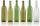Mrs. Violet brewed 15 l of raspberry juice. She poured it into 17 bottles. Some were 3/4 liter and some one liter. How many was bottles of this two types?
5. Largest angle of the triangleWhat is the largest angle of the triangle if the second angle is 10° greater than twice the first and the third is 30° smaller than the second?
6. EquationsSolve following system of equations: 6(x+7)+4(y-5)=12 2(x+y)-3(-2x+4y)=-44
7. Motel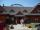Three different dinners A, B, C are served in the motel. Three 20 members went to motel. Group 1 ordered dinners: A 20 members and their average dinner price in the group was CZK 200. From Group 2, they ordered a A: 10 persons, B 10 dinners, and their aver
8. RewardThree workers have shared a common reward 13110 CZK follows: first worker got 35% less than the second and third worker got 20% more than the second worker. How much got each worker?
9. Barrel 4Barrel of water weighs 63 kg. After off 75% water, the weight of the barrel with water is 21 kg. How many kg weigh empty barrel and how many kgs water in it?
10. CuZn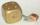Brass is an alloy of copper and zinc. The 10 centimeter brass cube has a weight of 8.6 kg. Copper density is 8930 kg/m3, the zinc density is 7130 kg/m3. Calculate how many kg of copper and zinc a cube contains.
11. Donuts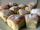In the dining room at one table sit Thomas, Filip and Martin. Together they eat 36 donuts. How many donuts eaten everyone, when Filip ate twice as much as Thomas and Martin even half over Filip?
12. Buttons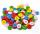Shirt has 6 buttons, blouse has 4 buttons. All buttons are 176. Shirts and blouses are together 36. How many are the shirts and blouses?
13. CoinsDenis and Zdeno together have 97 coins. If Denis had 4 coins less than he has now, the number of the coins would be in the ratio 14: 17. Determine the number of coins owned by Denis and Zdeno.
14. TheatroTheatrical performance was attended by 480 spectators. Women were in the audience 40 more than men and children 60 less than half of adult spectators. How many men, women and children attended a theater performance?
15. Two planes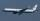Two planes flying from airports A and B, 420 km distant against each other. Plane from A took off 15 minutes later and flies at an average speed of 40 km/h higher than the plane from B. Determine the average speed of this two aircraft if you know that it
16. Men, women and childrenOn the trip went men, women and children in the ratio 2:3:5 by bus. Children pay 60 crowns and adults 150. How many women were on the bus when a bus was paid 4,200 crowns?
17. PotatoesDaniela and Michael would jointly dug potatoes for 7.5 hours. But if Daniela was working alone she would take 2.5 hours more as if he were working with Michael. Determine how much for the work done by Michael himself and how much Daniela herself.Number 118 divide into two addends, so first addend is 69 greater than 75% of the second addend.
19. Contestants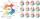In the competition it was possible to get 0-5 points. In fact, 5 contestants of the 15 best contestants has earned 5 points or 4 points (which have won 10 contestants). The number of contestants who earned 3 points was the same as the number of contestan
20. Three figures - numbersThe sum of three numbers, if each is 10% larger than the previous one, is 662. Determine the figures.

Do you have an interesting mathematical word problem that you can't solve it? Enter it, and we can try to solve it.

To this e-mail address, we will reply solution; solved examples are also published here. Please enter e-mail correctly and check whether you don't have a full mailbox.

Do you have a system of equations and looking for calculator system of linear equations?